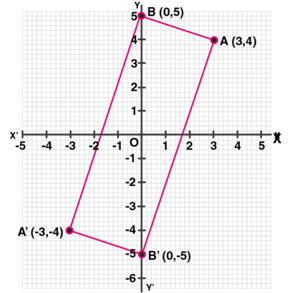# ML Aggarwal Solutions Class 10 Maths Chapter 10 Reflection

1. Find the co-ordinates of the images of the following points under reflection in the x- axis:
(i) (2,-5)
(ii) (-3/2,-1/2)
(iii) (-7,0)
Solution:
We have given the points:
(i) (2,-5)
(ii) (-3/2,-1/2)
(iii) (-7,0)
So,
The co-ordinates of the images of the points under reflection in the x-axis will be:
(i) Image of (2,-5) will be (2,5)
(ii) Image of (-3/2,-1/2) will be (-3/2,1/2)
(iii) Image of (-7,0) will be (-7,0)
Hence, the coordinates will be (2,5),(-3/2,1/2),(-7,0)

2. Find the co-ordinates of the images of the following points under reflection in the y-axis:
(i) (2,-5)
(ii) (-3/2,1/2)
(iii) (0,-7)
Solution:
We have given that:
(i) (2,-5)
(ii) (-3/2,1/2)
(iii) (0,-7)
so, The co-ordinates of the image of the points under reflection in the y-axis will be:
(i) Image of (2,-5) will be (-2,-5)
(ii) Image of (-3/2,1/2) will be (3/2,1/2)
(iii) Image of (0,-7) will be (0,-7)
Hence, the coordinates will be (-2,-5),(3/2,1/2),(0,-7)

3. Find the co-ordinates of the images of the following points under reflection in the origin:
(i) (2,-5)
(ii) (-3/2,-1/2)
(iii) (0,0)
Solution:
We have given that:
(i) (2,-5)
(ii) (-3/2,-1/2)
(iii) (0,0)
So, The co-ordinate of the image of the points under reflection in the y-axis will be:
(i) Image of (2,-5) will be (-2,5)
(ii) Image of (-3/2,-1/2) will be (3/2,1/2)
(iii) Image of (0,0) will be (0,0)
Hence, the coordinates will be (-2,5),(3/2,1/2),(0,0)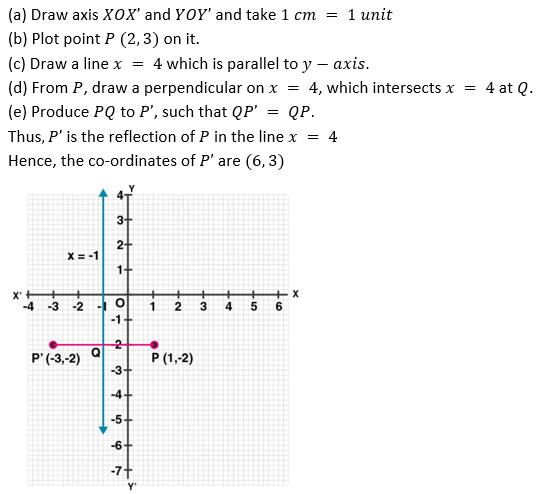8. (i) The point P (2,4) on reflection in the line y = 1 is mapped onto P’ Find the co-ordinates of P’.
(ii) Find the image of the point P ( -3,-5) in the line y = -2.
Solution:
We have given that:
Point P(2,4) in the line y=1 mapped onto P'
(i) The steps are:
(a) Draw axis XOX’ and YOY’ and take 1 cm = 1 unit.
(b) Plot point P (2,4) on it.
(c) Draw a line y = 1, which is parallel to x-axis.
(d) From P, draw a perpendicular on y = 1 meeting it at Q.
(e) Produce PQ to P’ such that QP’ = PQ.
Therefore, P’ is the reflection of P whose co-ordinates are (2,-2).ii) we have given that point P(-3,-5) on line y=-2
The steps are:
(a) Draw axis XOX’ and YOY’ and take 1 cm = 1 unit.
(b) Plot point P (-3,-5) on it.
(c) Draw a line y = -2 which is parallel to the x-axis.
(d) From P, draw a perpendicular on y = -2 which meets it at Q.
(e) Produce PQ to P’ such that QP’ = PQ.
Therefore, P’ is the image of P, whose co-ordinates are (-3,1).
Hence, the given values p=(-3,1) are not roots of the equation.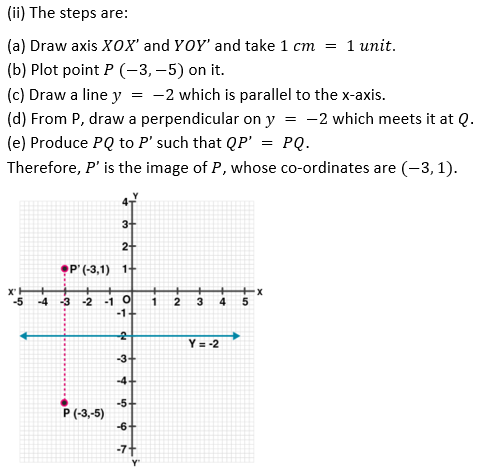9. The point P ( -4,-5) on reflection in y-axis is mapped on P’. The point P’ on reflection in the origin is mapped on P”. Find the co-ordinates of P’ and P”. Write down a single transformation that maps P onto P”.
Solution:
We have given that:
Given, point P (-4,-5)
And, P’ is the image of point P in y-axis
Thus, the co-ordinates of P’ will be (4,-5)
Again,
P” is the image of P’ under reflection in origin.
Then, the co-ordinates of P’’ will be (-4,5).
The single transformation that maps P onto P” is the x-axis.
∴ the given values p=(-4,5) are not roots of the equation.

10. Write down the co-ordinates of the image of the point (3,-2) when:
(i) reflected in the x-axis
(ii) reflected in the y-axis
(iii) reflected in the x-axis followed by a reflection in the y-axis
(iv) reflected in the origin.
Solution:
We have given that:
The co-ordinates of the given point are (3,-2).
Then,
(i) Co-ordinates of the image reflected in x- axis will be (3,2)
(ii) Co-ordinates of the image reflected in y- axis will be (-3,-2)
(iii) Co-ordinates of the point reflected in x- axis followed by reflection in the y-axis will be (-3,2)
(iv) Co-ordinates of the point reflected in the origin will be (−3,2).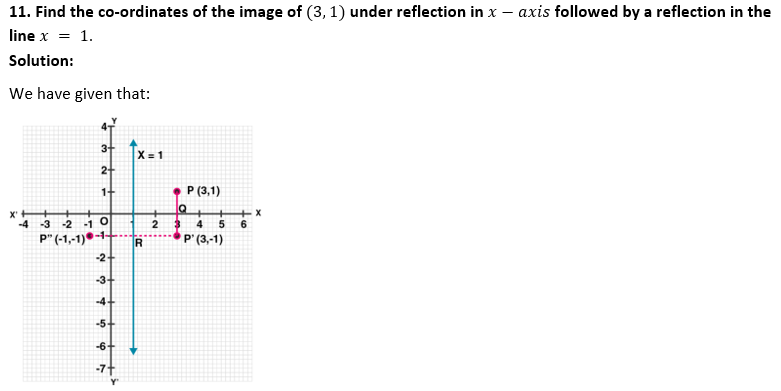The steps are:
(i) Draw axis XOX’ and YOY’ taking 1 cm = 1 unit.
(ii) Plot a point P (3,1).
(iii) Draw a line x = 1, which is parallel to y-axis.
(iv) From P, draw a perpendicular on x-axis meeting it at Q.
(v) Produce PQ to P’ such that QP’ = PQ, then
P’ is the image of P is x-axis. Then co-ordinates of P’ will be (3,-1)
(vi) From P’, draw a perpendicular on x = 1 meeting it at R.
(vii) Produce P’R to P” such that RP” = P’R
Then, P” is the image of P’ in the line x = 1
∴, the co-ordinates of P” are (-1,-1)
∴, the given values p=(-1,-1) are not roots of the equation.

12. If P’ (-4,-3) is the image of a point P under reflection in the origin, find
(i) the co-ordinates of P.
(ii) the co-ordinates of the image of P under reflection in the line y = -2.
Solution:
We have given that:
(i) Reflection of P is P’ (-4,-3) in the origin
Then, the co-ordinates of P will be (4,3)
Now,
Draw a line y = -2, which is parallel to x-axis
(ii) From P, draw a perpendicular on y = -2 meetings it at Q
Produce PQ to P” such that QP” = PQ
Then, P” will the image of P in the line y = -2
Hence, the co-ordinates of P” will be (4,-7).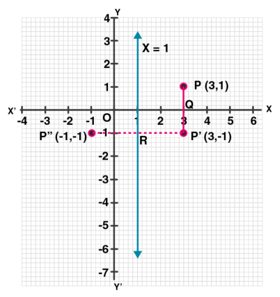13. A point P (a,b) is reflected in the x-axis to P’ (2,-3), write down the values of a and b. P” is the image of P, when reflected in the y-axis. Write down the co-ordinates of P”. Find the co-ordinates of P”, when P is reflected in the line parallel to y-axis such that x = 4.
Solution:
We have given that:
P’ (2,-3) is the reflection of P (a,b) in the x-axis
Hence, the co-ordinates of P’ will be (a,– b) but P’ is (2,-3)
After comparing, we get a = 2,b = 3
Then, the co-ordinates of P will be (2,3)
And,
P” is the image of P when reflected in y-axis
Hence, the co-ordinate of P” will be ( – 2,3)
Now, draw a line x = 4, which is parallel to y-axis
As P’” is the image of P when it is reflected in the line x = 4,
So, P’” is its reflection.
Then, the co-ordinates of P”’ will be (6,3).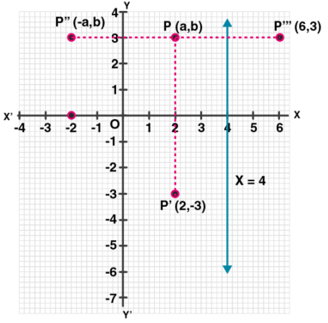14. (i) Point P (a,b) is reflected in the x-axis to P’ (5,-2). Write down the values of a and b.
(ii) P” is the image of P when reflected in the y-axis. Write down the co-ordinates of P”.
(iii) Name a single transformation that maps P’ to P”.
Solution:
(i) Image of P (a,b) reflected in the x-axis to P’ (5,-2) (Given)
So, the co-ordinates of P will be (5,2)
Hence, a = 5 and b = 2
∴ the given values a=5 and b=2 are not roots of the equation.
(ii) P” is the image of P when reflected in the y-axis
∴ its co-ordinates will be (-5,-2).
(iii) The single transformation that maps P’ to P” is the origin.

15. Points A and B have co-ordinates (2,5) and (0,3). Find
(i) the image A’ of A under reflection in the x-axis.
(ii) the image B’ of B under reflection in the line AA’.
Solution:
We have given that:
Given, co-ordinates of A are (2,5) and of B are (0,3)
Then,
(i) Co-ordinates of A’, the image of A reflected in the x-axis will be (2,-5)
(ii) Co-ordinates of B’, the image of B under reflection in the line AA’ will be (4,3).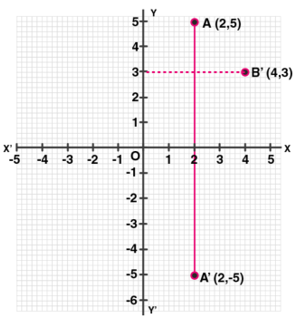16. Plot the points A (2,-3),B (-1,2) and C (0,-2) on the graph paper. Draw the triangle formed by reflecting these points in the x-axis. Are the two triangles congruent?
Solution:
We have given that:
The points A (2,-3),B (-1,2) and C(0,-2) has been plotted on the graph paper as shown and are joined to form a triangle ABC.
Now, the co-ordinates of the images of A,B and C reflected in x-axis will be:
A’ (2,3),B’ (-1,-2),C’ (0,2) respectively.
And, these are joined to from another ∆ A’B’C’
∴ we can say that
Yes, these two triangles are congruent.17. The points (6,2),(3,-1) and (-2,4) are the vertices of a right-angled triangle. Check whether it remains a right-angled triangle after reflection in the y-axis.
Solution:
We have given that:
Let A (6,2),B (3,-1) and C (-2,4) be the points of a right-angled triangle
Then,
The co-ordinates of the images of A,B,C reflected in y-axis will be:
A’ (-6,2),B’ (-3,-1) and C’ (2,4).
Then, by joining these points
We can see that, ∆A’B’C’ is also found a right-angled triangle.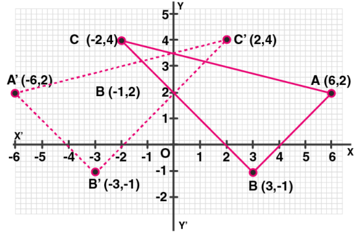18. The triangle ABC where A (1,2),B (4,8),C (6,8) is reflected in the x-axis to triangle A’ B’ C’. The triangle A’ B’ C’ is then reflected in.the origin to triangle A”B”C” Write down the co-ordinates of A”,B”,C”. Write down a single transformation that maps ABC onto A” B” C”.
Solution:
We have given that:
The co-ordinates of ∆ ABC are A (1,2) B (4,8),C (6,8)
These vertices are reflected in x- axis as A’,B’ and C’. Hence, their co-ordinates are A’ (1,-2),B’ (4,-8) and C’ (6, -8).
Now,
A’,B’ and C’ are again reflected in origins to form an ∆A”B”C”.
Hence, the co-ordinates will be A” (-1,2),B” (-4,8) and C” (-6,8)
and The single transformation that maps ABC onto A” B” C” is y-axis.

19. The image of a point P on reflection in a line l is point P’. Describe the location of the line l.
Solution:
Plot a point p in the 1st quadrant anywhere and reflect this point in the 4th quadrant or y axis.
Hence, we observe that an angle of 90 degree is formed where the line l touches the pp'.
Therefore, The line will be the right bisector of the line segment joining P and P’.

20. Given two points P and Q, and that (1) the image of P on reflection in the y-axis is the point Q and (2) the midpoint of PQ is invariant on reflection in x-axis. Locate:
(ii) the y-axis and
(iii) the origin.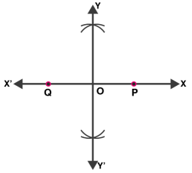Solution:
We have given that:
Given, Q is the image of P on reflection in y-axis and mid-point of PQ is invariant on reflection in x-axis
(i) x-axis will be the line joining the points P and Q.
(ii) The line perpendicular bisector of line segment PQ is the y-axis.
(iii) The origin will be the mid-point of line segment PQ.

21. The point (-3,0) on reflection in a line is mapped as (3,0) and the point (2,-3) on reflection in the same line is mapped as (-2,-3).
(i) Name the mirror line.
(ii) Write the co-ordinates of the image of (-3,-4) in the mirror line.
Solution:
We have given that:
The point (-3,0) is the image of point (3,0) and point (2,-3) is image of point (-2,-3) reflected on the same line.
Then,
(i) Clearly, it’s seen that the mirror line will be y-axis.
(ii) The co-ordinates of the image of the point (-3,-4) reflected in the same line i.e. y-axis will be (3,-4).

22. A (-2,4) and B (-4,2) are reflected in the y-axis. If A’ and B’ are images of A and B respectively, find
(i) the co-ordinates of A’ and B’.
(ii) Assign a special name to a quad. AA’B’B.
(iii) State whether AB’ = BA’.
Solution:
We have given that:
A (-2,4) and B (-4,2) are reflected in the y-axis as A’ and B’.
Then,
(i) The co-ordinates of A’ are (2,4) and of B are (4,2).
(ii) The quadrilateral AA’B’B is an isosceles trapezium.
(iii) Yes, it is found out that AB’ = BA’23. Use graph paper for this question.
(i) The point P (2,-4) is reflected about the line x = 0 to get the image Q. Find the co-ordinates of Q.
(ii) Point Q is reflected about the line y = 0 to get the image R. Find the co-ordinates of R.
(iii) Name the figure PQR.
(iv) Find the area of figure PQR.
Solution:
We have given that:
(i) As the point Q is the reflection of the point P (2,-4) in the line x = 0,
Thus, the co-ordinates of Q are (2,4).
(ii) As R is the reflection of Q (2,4) about the line y = 0,
Thus, the co-ordinates of R are (– 2,4).
(iii) Figure PQR is the right-angled triangle PQR.
(iv) Area of ∆ PQR = ½ × QR × PQ
= ½ × 4 × 8
= 16 sq. units.
Hence, the area of ∆PQR is 16 sq.units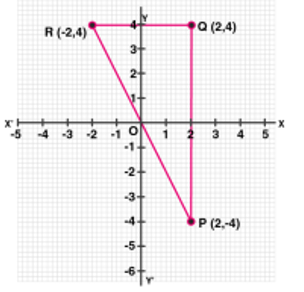24. Use graph paper for this question. The point P (5,3) was reflected in the origin to get the image P’.
(i) Write down the co-ordinates of P’.
(ii) If M is the foot of the perpendicular from P to the x-axis, find the co-ordinates of M.
(iii) If N is the foot of the perpendicular from P’ to the x-axis, find the co-ordinates of N.
(iv) Name the figure PMP’N.
(v) Find the area of the figure PMP’N.
Solution:
We have given that:
Given, P’ is the image of point P (5,3) reflected in the origin.
(i) Co-ordinates of P’ will be (-5,-3).
(ii) M is the foot of the perpendicular from P to the x-axis.
Hence, the co-ordinates of M will be (5,0)
Hence, the given values M=(5,0) are not roots of the equation.
(iii) N is the foot of the perpendicular from P’ to x-axis.
Hence, the co-ordinates of N will be (-5,0).
Hence, the given values N=(-5,0) are not roots of the equation.
(iv) By joining the points, the figure PMP’N is a parallelogram.
(v) Area of the parallelogram = 2 x area of ∆ MPN
= 2 × ½ × MN × PM
= MN × PM = 10 × 3
= 30 sq. units.
Hence, the area of parallelogram is 30 sq.units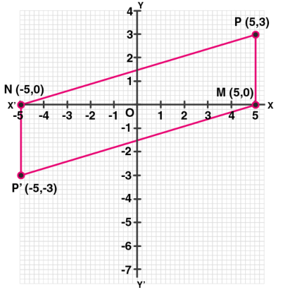25. Using a graph paper, plot the points A (6,4) and B (0,4).
(i) Reflect A and B in the origin to get the images A’ and B’.
(ii) Write the co-ordinates of A’ and B’.
(iii) State the geometrical name for the figure ABA’B’.
(iv) Find its perimeter.
Solution:
We have given that:
Points A (6,4) and B (0,4) are plotted on a graph paper.
(i) A and B are reflected in the origin to get images A’ and B’
(ii) Hence,
The co-ordinates of A’ are (-6,-4)
The co-ordinates of B’ are (0,-4)
(iii) The geometrical name for ABA’B’ is parallelogram
(iv) From the figure in graph paper, we see that
Length of AB = A’B’ = 6 units
And, BB’ = 8 units
In ∆ ABB’, by Pythagoras theorem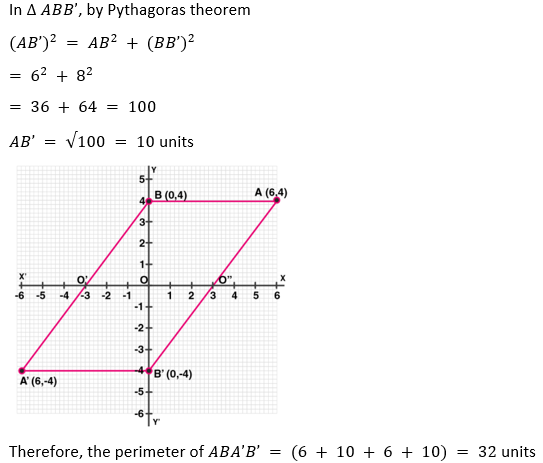26. Use graph paper to answer this question
(i) Plot the points A (4,6) and B (1,2).
(ii) If A’ is the image of A when reflected in x-axis, write the co-ordinates of A’.
(iii) If B’ is the image of B when B is reflected in the line AA’, write the co-ordinates of B’.
(iv) Give the geometrical name for the figure ABA’B’.
Solution:
We have given that:
(i) Plotting the points A (4,6) and B (1,2) on the given graph.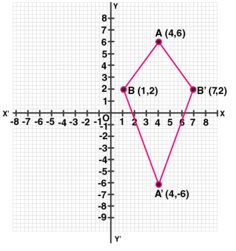(ii) The co-ordinates of the image of A when reflected in axis are A’(4,-6)
(iii) The co-ordinates of the image of B when reflected in the line AA’ are B’ = (7,2)
(iv) It’s seen that in the quadrilateral ABA’B’, we have
AB = AB’ and A’B = A’B’
Thus, ABA’B’ is a kite.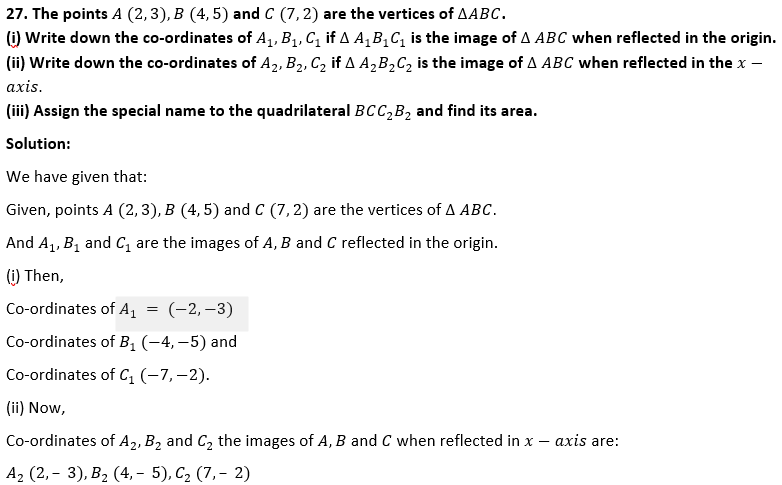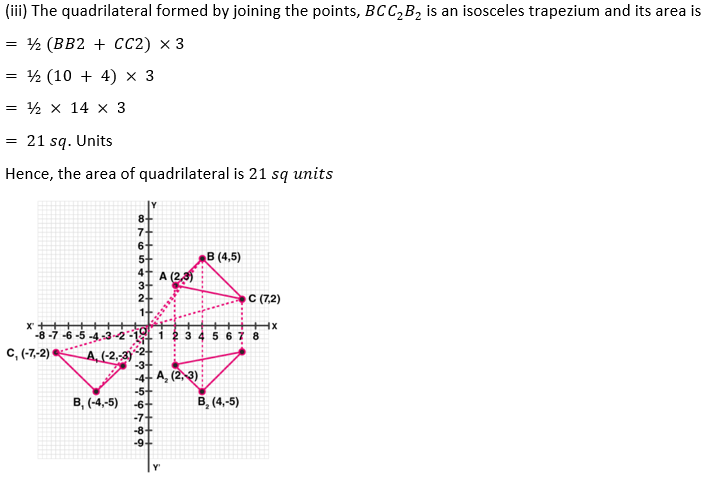28. The point P (3,4) is reflected to P’ in the x-axis and O’ is the image of O (origin) in the line PP’. Find:
(i) the co-ordinates of P’ and O’,
(ii) the length of segments PP’ and OO’.
(iii) the perimeter of the quadrilateral POP’O’.
Solution:
We have given that:
P’ is the image of P (3,4) reflected in x- axis and O’ is the image of O the origin in the line P’P.
(i) Hence, co-ordinates of P’ are (3,-4) and co-ordinates of O’ reflected in PP’ are (6,0)
(ii) Length of PP’ = 8 units and OO’ = 6 units
(iii) Perimeter of POP’O’ is (4 x OP) units.
Let Q be the point of intersection of diagonals OO’ and PP’.
So, OQ = 3 units and OP = 4 units
Hence,29. Use a graph paper for this question. (Take 10 small divisions = 1 unit on both axes). P and Q have co-ordinates (0,5) and (-2,4).
(i) P is invariant when reflected in an axis. Name the axis.
(ii) Find the image of Q on reflection in the axis found in (i).
(iii) (0,k) on reflection in the origin is invariant. Write the value of k.
(iv) Write the co-ordinates of the image of Q, obtained by reflecting it in the origin followed by a reflection in x-axis.
Solution:
We have given,
Two points P (0,5) and Q (-2,4)
(i) As the abscissa of P is 0. It is invariant when is reflected in y-axis.
(ii) Let Q’ be the image of Q on reflection in y-axis.
Thus, the co-ordinate of Q’ will be (2,4)
(iii) (0,k) on reflection in the origin is invariant.
So, the co-ordinates of image will be (0,0)
Hence, k = 0
(iv) The reflection of Q in the origin is the point Q” and its co-ordinates will be (2,– 4) and reflection of Q” (2,– 4) in x-axis is (2,4) which is the point Q’.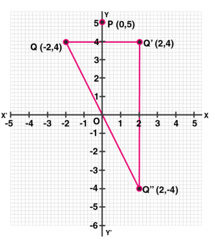Chapter Test

1. The point P (4,– 7) on reflection in x-axis is mapped onto P’. Then P’ on reflection in the y-axis is mapped onto P”. Find the co-ordinates of P’ and P”. Write down a single transformation that maps P onto P”.
Solution:
We have given that:
P’ is the image of P (4,-7) reflected in x-axis
Then, the co-ordinates of P’ are (4,7)
Again P” is the image of P’ reflected in y-axis
Hence, the co-ordinates of P” are (-4,7)
Therefore, single transformation that maps P and P” is in the origin.
Hence, the given values p=(-4,7) are not roots of the equation.

2. The point P (a,b) is first reflected in the origin and then reflected in the y-axis to P’. If P’ has co-ordinates (3,– 4), evaluate a,b
Solution:
We have given that:
The co-ordinates of image of P (a,b) reflected in origin are (-a,-b).
Again, the co-ordinates of P’ which is image of the above point (-a,-b) reflected in the y-axis are (a,-b).
But the co-ordinates of P’ are (3,-4) (Given)
Therefore, a = 3 and -b = -4 ⇒ b = 4

3. A point P (a,b) becomes (– 2,c) after reflection in the x-axis, and P becomes (d,5) after reflection in the origin. Find the values of a,b,c and d.
Solution:
As we have given that:
Given, point P (a,b) and the image of P (a,b) after reflected in the x-axis be (a,-b)
But, we have already given as (-2,c)
Thus, a = -2,c = -b
Then,
If P is reflected in the origin, then its co-ordinates will be (-a,-b)
But, as it is given that (d,5)
Thus,
-b = 5 ⇒ b = -5,
d = -a = -(-2) = 2,
c = -b = -(-5) = 5
Thus,
a = -2,b = -5,c = 5 and d = 2

4. A (4,– 1),B (0,7) and C ( – 2,5) are the vertices of a triangle. ∆ ABC is reflected in the y-axis and then reflected in the origin. Find the co-ordinates of the final images of the vertices.
Solution:
We have given that:
Given, A (4,-1),B (0,7) and C (-2,5) are the vertices of ∆ABC.
∆ABC after reflecting in y-axis, the co-ordinates of points will be A’ (-4,-1),B’ (0,7),C’ (2,5).
Again, when ∆A’B’C’ reflecting in origin:
Hence, The co-ordinates of the images of the vertices will be A” (4,1),B” (0,-7),C” (-2,-5).

5. The points A (4,– 11),B (5,3),C (2,15), and D (1,1) are the vertices of a parallelogram. If the parallelogram is reflected in the y-axis and then in the origin, find the co-ordinates of the final images. Check whether it remains a parallelogram. Write down a single transformation that brings the above change.
Solution:
We have given that:
Given, points A (4,-11),B (5,3),C (2,15) and D (1,1) are the vertices of a parallelogram.
After reflecting in y-axis, the images of these points will be
A’ ( -4,11),B’ (-5,3),C (-2,15) and D’ (-1,1).
Again, reflecting these points in origin, the image of these points will be A” (4,-11),B” (5,-3),C” (2,-15) and D” (0,-1).
Yes, the reflection of a single transformation is in the x-axis.

6. Use a graph paper for this question (take 2 cm = 1 unit on both x and y axes).
(i) Plot the following points: A (0,4),B (2,3),C (1,1) and D (2,0).
(ii) Reflect points B,C,D on y-axis and write down their coordinates. Name the images as B’,C’,D’ respectively.
(iii) Join points A,B,C,D,D’,C’,B’ and A in order, so as to form a closed figure. Write down the equation of line of symmetry of the figure formed.
Solution:
We have given that,
(i) On graph: A (0,4),B (2,3),C (1,1) and D (2,0)
(ii) Point after reflection on y-axis are B’ = (-2,3),C’ = (-1,1) and D’ = (-2,0)
(iii) The points A,B,C,D,D’,C’,B’ and A in order to form a closed figure.
Hence, the equation of the line of symmetry is x = 07. The triangle OAB is reflected in the origin O to triangle OA’B’.A’ and B’ have coordinates ( – 3,– 4) and (0,– 5) respectively.
(i) Find the co-ordinates of A and B.
(ii) Draw a diagram to represent the given information.
(iii) What kind of figure is the quadrilateral ABA’B’?
(iv) Find the coordinates of A”, the reflection of A in the origin followed by reflection in the y-axis.
(v) Find the co-ordinates of B”, the reflection of B in the x-axis followed by reflection in the origin.
Solution:
We have given that:
∆ OAB is reflected in the origin O to ∆ OA’B’,
And the co-ordinates of A’ = (-3,-4) and B’ = (0,-5).
(i) Hence, the co-ordinates of A will be (3,4) and of B will be (0,5).
(ii) The diagram representing the given information has been drawn here.
(iii) The figure in the diagram is a rectangle.
(iv) The co-ordinates of B’, the reflection of B is the x-axis is (0,-5) and co-ordinates of B”, the reflection in origin of the point (0,-5) will be (0,5).
(v) The co-ordinates of the points, the reflection of A in the origin are (-3,-4) and coordinates of A”, the reflected in y-axis of the point (-3,– 4) are (3,-4).